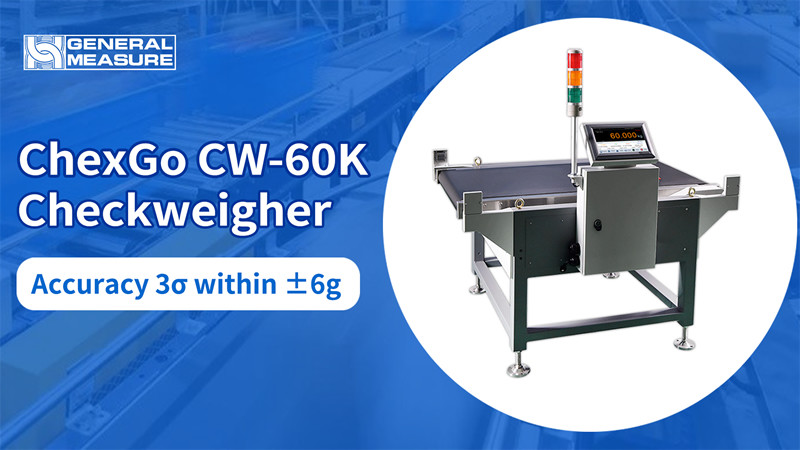## Best Performance: Precision within ±6g with General Measure Heavy Load Checkweigher ChexGo CW-60KGeneral Measure Technology Co. Ltd. (China) - Press Release: CW-60K is the dynamic checkweigher with 60kg’s weight range. This heavy load checkweigher’s accuracy before was tested as ±10g, which was to simply record the error value during the loop test. However, for obtaining the more accurate data, we introduced the concept of 3σ. After testing and calculating, the maximum accuracy of 60kg large-scale checkweigher is within ±6g, which has both theoretical and experimental support.

In a measurement event that conforms to a normal distribution, σ describes the degree of dispersion of the data distribution. The smaller the σ, the more concentrated the data distribution, vice versa. 3σ is usually used as the maximum accuracy for checkweigher. The 3σ calculated through experimental tests followed by the formula can better reflect the accuracy and repeatability of the checkweigher.Under the guidance of professionals, we began to test CW-60K cyclically. Two test objects with 60008g and 60006g were tested 100 times separately. The weight and error value of each time weighing were recorded. The experimental test data is slightly influenced by uncontrollable factors, such as temperature, humidity, wind speed and wind direction, ground inclination, etc., and the influence of these small factors on the error conforms to the normal distribution. So the weighing results for 100 times also conform to the normal distribution. Therefore, according to the formula, the standard deviation σ is calculated as 1.0g (test object is 60008g) and 1.8g (test object is 60006g), and 3σ is 2.9 g and 5.4g, respectively.Distribution diagram of 100 times testing of weight data (Object: 60008g)Distribution diagram of 100 times testing of weight data (Object: 60006g)After calculating the values of σ and 3σ, we found there is 99.7% possibility that 3σ will be within the range of ± 2.9g and ± 5.4g. Then, we have tested the objects of 45kg, 25kg, 10kg and 1kg respectively, and the results are shown in the below figure:Following the same testing and calculation, there is 99.7% possibility that 3σ will be within the range showing on above’s table. In real life, it is often considered to be the impossible event. Therefore, the maximum accuracy of our large-scale checkweigher with 60kg is less than 6g, but the accuracy will also vary depending on the weight of the object, size and other factors.In the comparison with our competitors, we found that the accuracy of a large scale’s checkweigher controlled within ± 6g is better than most Chinese brand checkweigher and comparable to internationally renowned brands. Therefore, we are very proud to introduce CW60K, the 60kg large-scale checkweigher with high precision.

We offer:

• OEM and ODM on Checkweigher
• Dynamic Checkweigher Controller

Other articles from General Measure Technology Co. Ltd..

© General Measure Technology Co. Ltd. / International Weighing Review